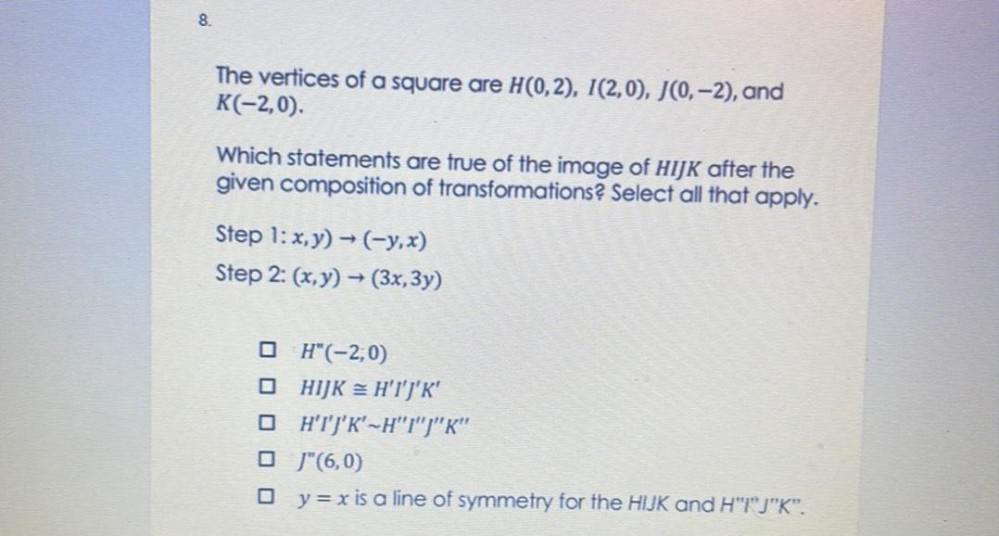Question:

# The vertices of a square are H(0,2), I(2,0), J(0-2), and K(-2,0). Which statements are true of the image of HIJK after the given composition of transformations? Select all that apply. Step 1: x,y) → (The vertices of a square are H(0,2), I(2,0), J(0-2), and K(-2,0). Which statements are true of the image of HIJK after the given composition of transformations? Select all that apply. Step 1: x,y) → (-y,x) Step 2: (x,y) → (3x, 3y) a)H"(-2,0) b) HIJK = H'I'J'K' c)H'I'J'K'~H"T")"K" d)J"(6,0) e)y= x is a line of symmetry for the HIJK and H"1"J"K".